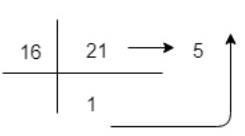Hexadecimal Number System is one the type of Number Representation techniques, in which there value of base is 16. That means there are only 16 symbols or possible digit values, there are 0, 1, 2, 3, 4, 5, 6, 7, 8, 9, A, B, C, D, E, F. Where A, B, C, D, E and F are single bit representations of decimal value 10, 11, 12, 13, 14 and 15 respectively. It requires only 4 bits to represent value of any digit. Hexadecimal numbers are indicated by the addition of either an 0x prefix or an h suffix.

Position of every digit has a weight which is a power of 16. Each position in the Hexadecimal system is 16 times more significant than the previous position, that means numeric value of an hexadecimal number is determined by multiplying each digit of the number by the value of the position in which the digit appears and then adding the products. So, it is also a positional (or weighted) number system.

Each Hexadecimal number can be represented using only 4 bits, with each group of bits having a distich values between 0000 (for 0) and 1111 (for F = 15 = 8+4+2+1). The equivalent binary number of Hexadecimal number are as given below.

 Hex digit 1 0 2 3 4 5 6 7 Binary 0000 0001 0010 0011 0100 0101 0110 0111

 Hex digit 8 9 A = 10 B = 11 C = 12 D = 13 E = 14 F = 15 Binary 1000 1001 1010 1011 1100 1101 1110 1111

Hexadecimal number system is similar to Octal number system. Hexadecimal number system provides convenient way of converting large binary numbers into more compact and smaller groups.

Most Significant Bit (MSB)Hex PointLeast Significant Bit (LSB)
16216116016-116-216-3
2561611/161/2561/4096

Since base value of Hexadecimal number system is 16, so there maximum value of digit is 15 and it can not be more than 15. In this number system, the successive positions to the left of the hexadecimal point having weights of 160, 161, 162, 163and so on. Similarly, the successive positions to the right of the hexadecimal point having weights of 16-1, 16-2, 16-3and so on. This is called base power of 16. The decimal value of any hexadecimal number can be determined using sum of product of each digit with its positional value.

Example-1 − The number 512 is interpreted as

512=2x162+0x161+0x160=200

Here, right most bit 0 is the least significant bit (LSB) and left most bit 2 is the most significant bit (MSB).

Example-2 − The number 2015.0625 is interpreted as

2015.0625=7x162+13x161+15x160+1x16-1=7DF.10

Here, right most bit 0 is the least significant bit (LSB) and left most bit 7 is the most significant bit (MSB).

Example-3 A a decimal number 21 to represent in Hexadecimal representation(21)10=1x161+5x160=(15)16
So, decimal value 21 is equivalent to 15 in Hexadecimal Number System.

## Applications of Hexadecimal Number System

Hexadecimal Number System is commonly used in Computer programming and Microprocessors. It is also helpful to describe colors on web pages. Each of the three primary colors (i.e., red, green and blue) is represented by two hexadecimal digits to create 255 possible values, thus resulting in more than 16 million possible colors. Hexadecimal number system is used to describe locations in memory for every byte. These hexadecimal numbers are also easier to read and write than binary or decimal numbers for Computer Professionals.